# Independent and Dependent Voltage and Current Sources

In electrical and electronic circuits, some components are used to supply the required electrical energy for the operation of the circuit. These components are known as energy sources. Energy sources are the active circuit elements that can supply the necessary excitation, i.e. required electrical energy, to the circuit. In practice, we have several types of voltage and current sources such as batteries, generators, alternators, photovoltaic cells, etc. In this article, we will discuss the different types of energy sources.

## Types of Energy Sources

The energy sources are broadly classified into the following two types −

• Voltage Sources
• Current Sources

An energy that is used to create a voltage (potential difference) between two points in an electric circuit is referred to as a voltage source. While, the energy source that delivers a specified electric current to the circuit is referred to as a current source.

Both voltage sources and current sources are further classified into the following two categories −

• Independent Sources
• Dependent Sources

Let us discuss the independent and dependent sources in detail one by one.

## Independent Voltage Source

A voltage source whose output voltage does not depend upon the voltage or current of any other part of the circuit is known as an independent voltage source. In other words, the independent voltage source is one whose output voltage is not affect by the voltage or current of any other part of the circuit.

The independent voltage sources may be classified into the following two types −

### Constant Voltage Sources

The constant voltage sources, also called time-invariant voltage sources, are those independent voltage sources whose output voltage remains constant throughout their operation. Examples of constant independent voltage sources are cells, batteries, and many other direct voltage sources.

### Time Variant Voltage Sources

The types of independent voltage sources whose output voltage changes with time are known as time-variant independent voltage sources. Although, it is important to note that the change in the output voltage of the time-variant independent voltage source does not occur due to change in the voltage or current of any other part of the circuit. But, it only changes with time. Examples of time-variant voltage sources are alternator (AC generator).

## Independent Current Source

The type of current source whose output current does not depend upon the voltage or current of any other part of the circuit is known as independent current source.

Similar to independent voltage sources, the independent current sources are also classified into two types −

### Constant Current Source

An independent current sources whose output current remains constant throughout its operation is known as a constant independent current source. It is also known as timeinvariant current source.

### Time-Variant Current Source

An independent current source whose output current changes time is referred to as a timevariant independent current source. Here, it must note that the output current does not change due to variation in voltage or current in any other part of the circuit, but it only changes with time.

Independent voltage and current sources may also be classified into the following categories, depending their internal resistance −

• Ideal Voltage Source
• Practical Voltage Source
• Ideal Current Source
• Practical Current Source

## Ideal Voltage Source

A voltage source which has zero internal resistance is known as ideal voltage source. Therefore, for an ideal voltage source, the output voltage always remains constant and equal to the EMF of the source. It is because, there is no voltage drop inside the source when current drawn from it. However, the ideal voltage sources do not exist in practice. These are just theoretical models of voltage sources.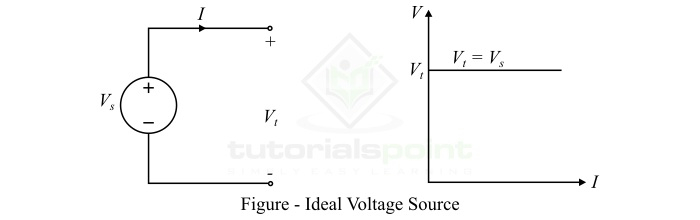## Practical Voltage Source

An independent voltage source which has a finite internal resistance is known as a practical voltage source or real voltage source. In a real voltage source, the internal resistance is shown connected in series with the source voltage as shown in the figure.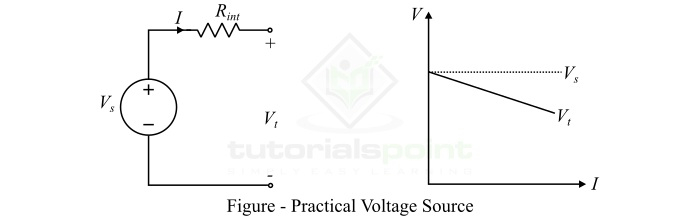Another important fact to note about the real voltage is that the output voltage or terminal voltage of the real voltage source decreases with the increase in current drawn from it when a load circuit is connected across its terminals. It is because, when a current is drawn from the source, it causes a voltage drop in the internal resistance. Consequently, the output voltage at the source terminals is reduced. The voltage sources such as cells, batteries, generator, etc. are examples of real voltage sources.

## Ideal Current Source

A current source which has infinite internal resistance is known as ideal current source. Therefore, the output current of an ideal current source is equal to the source current due to its infinite internal resistance. Thus, an ideal current source always delivers same current throughout its operation when a load is connected to it. Any change in the load circuit does not affect the output current of the ideal current source. Again, the ideal current sources are not practically possible.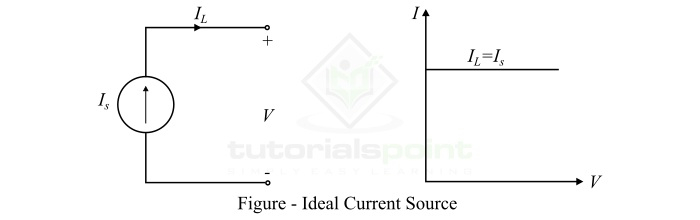## Practical Current Source

A practical current source (or real current source) is one which has a finite internal resistance which is connected in parallel with the source current. Therefore, when there is any change in the load voltage, the current delivered by the real current source is changed. It is because, the increase in the load voltage forces an extra current to flow through the internal resistance of the source, which results in the decreased output current.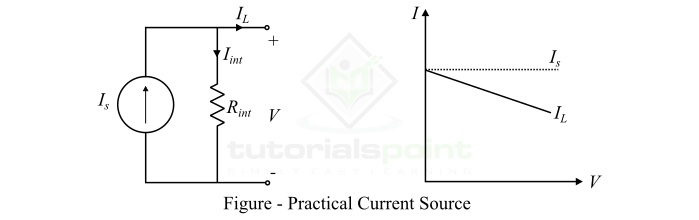Examples of real current sources include semiconductor devices such as diodes, transistors, operational amplifiers, etc.

## Dependent Sources

Dependent sources are those whose output voltage is governed by a voltage or current in any other part of the circuit. Dependent sources are represented using diamond shapes as shown in the figure below −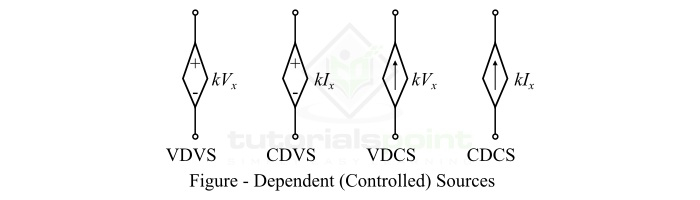Based on the combination of controlling quantity and output quantity, the dependent sources are classified into the following four types −

• Voltage Dependent Voltage Source (VDVS)
• Current Dependent Voltage Source (CDVS)
• Voltage Dependent Current Source (VDCS)
• Current Dependent Current Source (CDCS)

Sometimes, the dependent sources are referred to as controlled sources. The dependent sources are used in modeling the behavior of electronic devices such as amplifiers.

## Conclusion

From the above discussion, we can conclude that the voltage and current sources are the essential parts of electrical and electronic circuits. These sources provide the necessary energy for the excitation of the circuit. Both voltage and current sources are categorized as active circuit elements because they deliver power to the circuit.